#Algebra 2 Worksheets

Here is a graphic preview for all of the Radical Functions Worksheets. You can select different variables to customize these Radical Functions Worksheets for your needs. The Radical Functions Worksheets are randomly created and will never repeat so you have an endless supply of quality Radical Functions Worksheets to use in the classroom or at home. Our Radical Functions Worksheets are free to download, easy to use, and very flexible.

These Radical Functions Worksheets are a good resource for students in the 8th Grade through the 12th Grade.

Click the image to be taken to that Radical Functions Worksheets.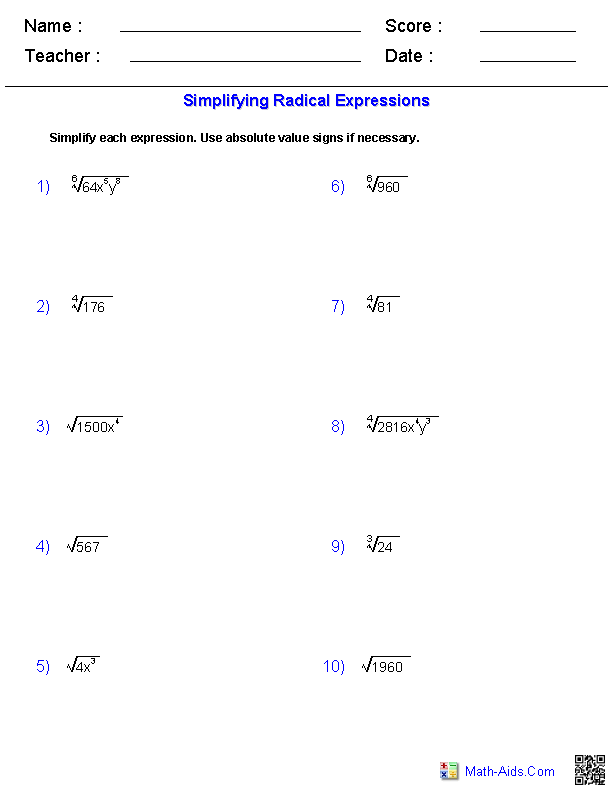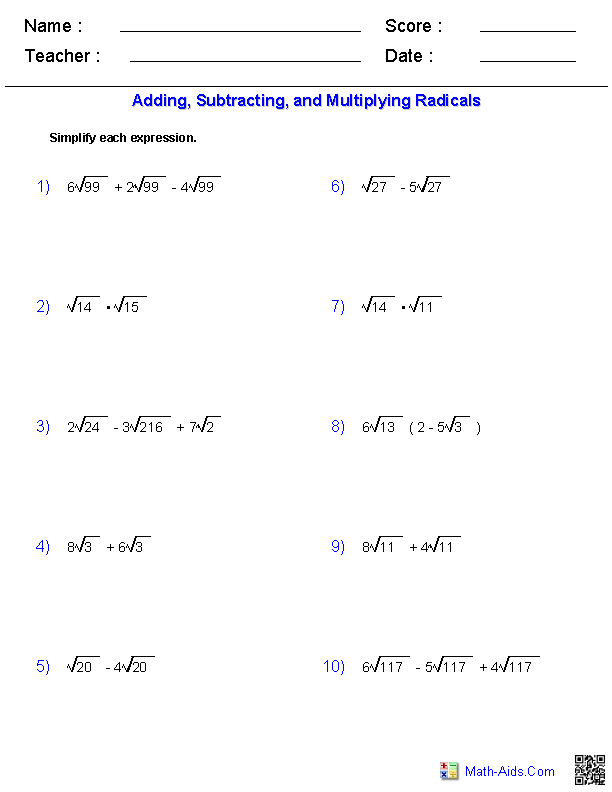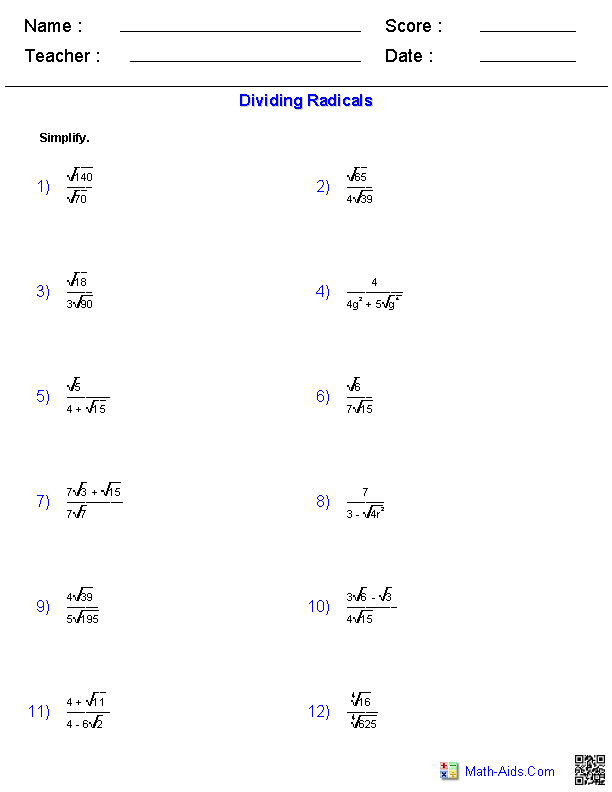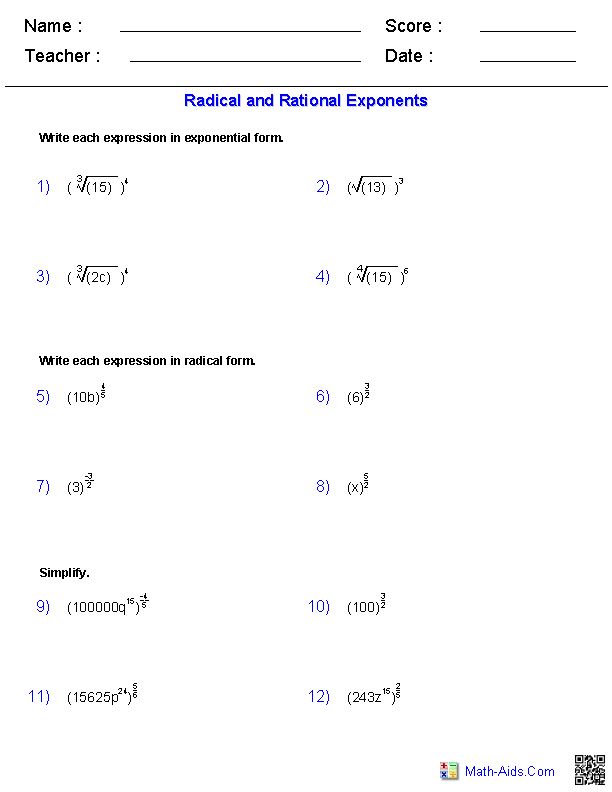##### Simplifying Rational ExponentsRadical Functions Worksheets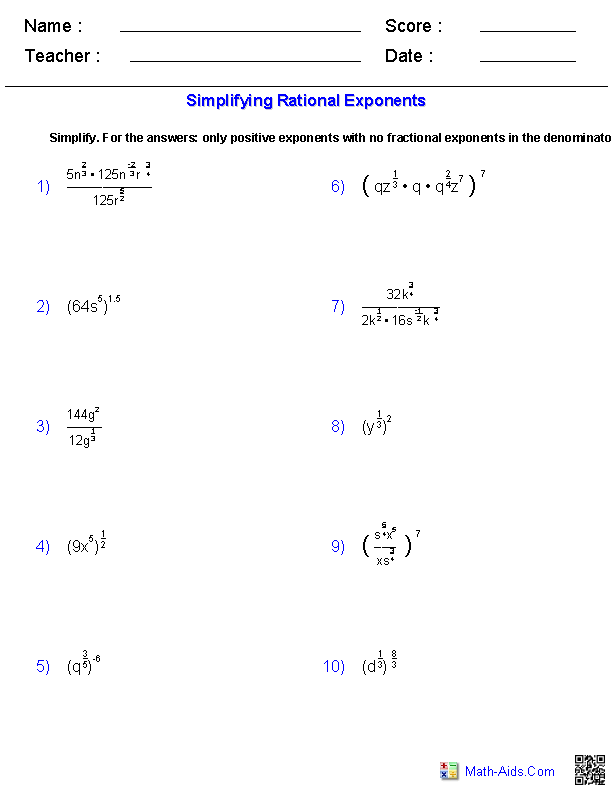##### Square Root EquationsRadical Functions Worksheets##### Rational Exponent EquationsRadical Functions Worksheets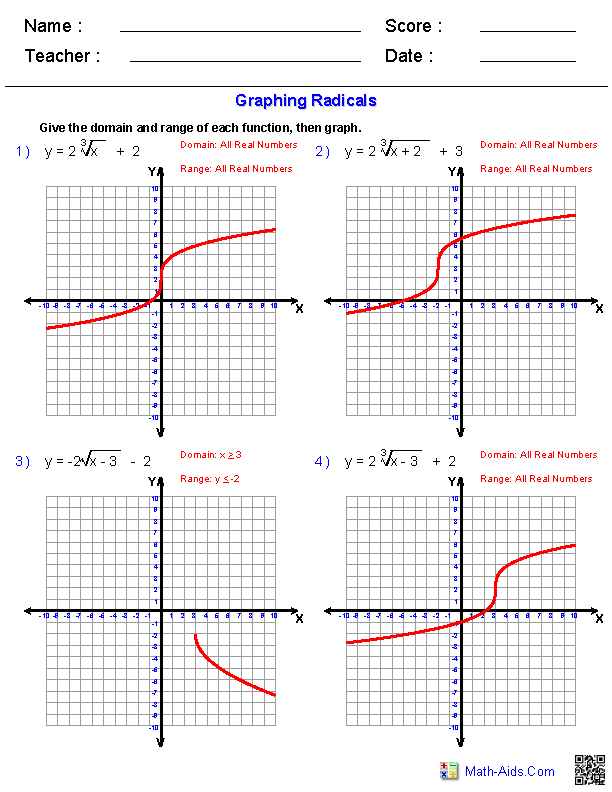Recommended Videos

## Description For All Radical Functions Worksheets

This radical functions worksheet will produce problems for simplifying radical functions. You may select the degree of the root function and whether to include variables or not. This radical functions worksheet is a good resource for students in the 9th Grade, 10th Grade, 11th Grade, and 12th Grade.

This radical functions worksheet will produce problems for Operations with Radical Functions. You may select the difficulty of the problems. This radical functions worksheet is a good resource for students in the 9th Grade, 10th Grade, 11th Grade, and 12th Grade.

This radical functions worksheet will produce problems for dividing radical expressions. You may select the difficulty of the problems. This radical functions worksheet is a good resource for students in the 9th Grade, 10th Grade, 11th Grade, and 12th Grade.

This radical functions worksheet will produce problems for radical and rational exponents. You may select whether to include variables or not. This radical functions worksheet is a good resource for students in the 9th Grade, 10th Grade, 11th Grade, and 12th Grade.

Simplifying Rational Exponents Worksheets
This radical functions worksheet will produce problems for simplifying rational exponents. You may select the difficulty of the problems. This radical functions worksheet is a good resource for students in the 9th Grade, 10th Grade, 11th Grade, and 12th Grade.

Square Root Equations Worksheets
This radical functions worksheet will produce problems for square root equations. You may select the difficulty of the problems. This radical functions worksheet is a good resource for students in the 9th Grade, 10th Grade, 11th Grade, and 12th Grade.

Rational Exponent Equations Worksheets
This radical functions worksheet will produce problems for rational exponent equations. You may select the difficulty of the problems. This radical functions worksheet is a good resource for students in the 9th Grade, 10th Grade, 11th Grade, and 12th Grade.